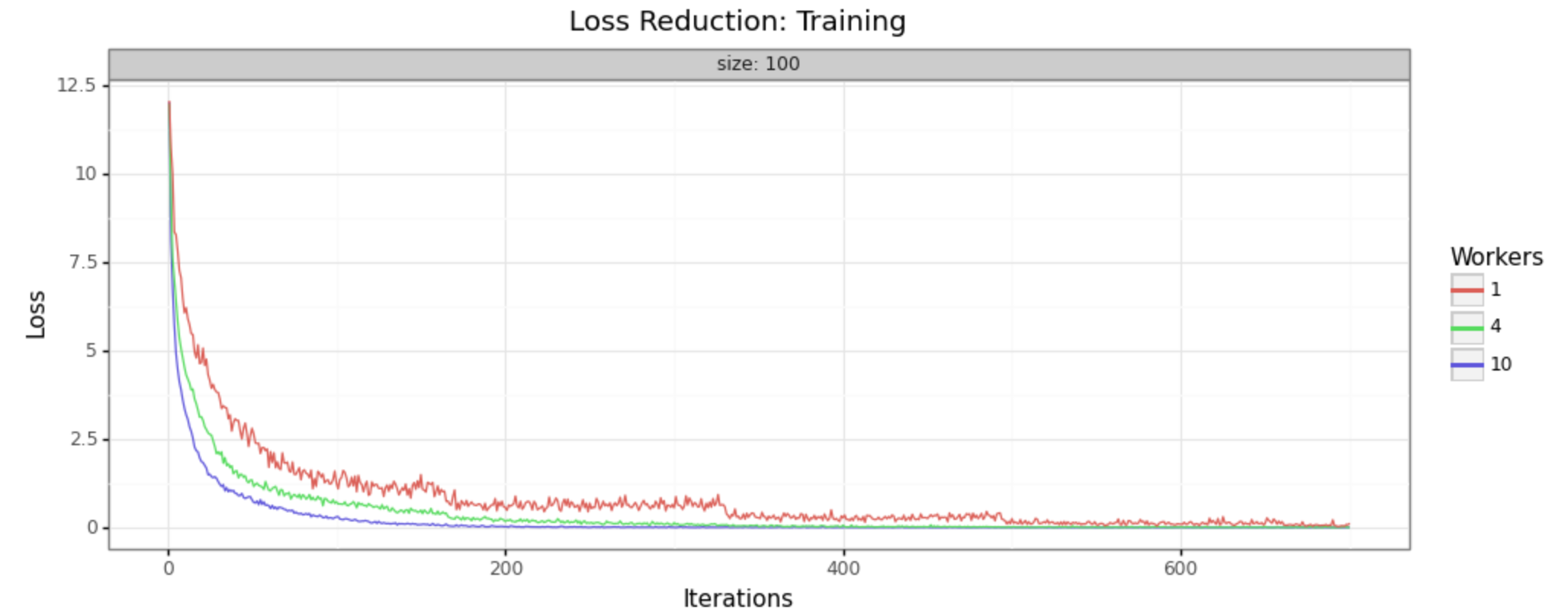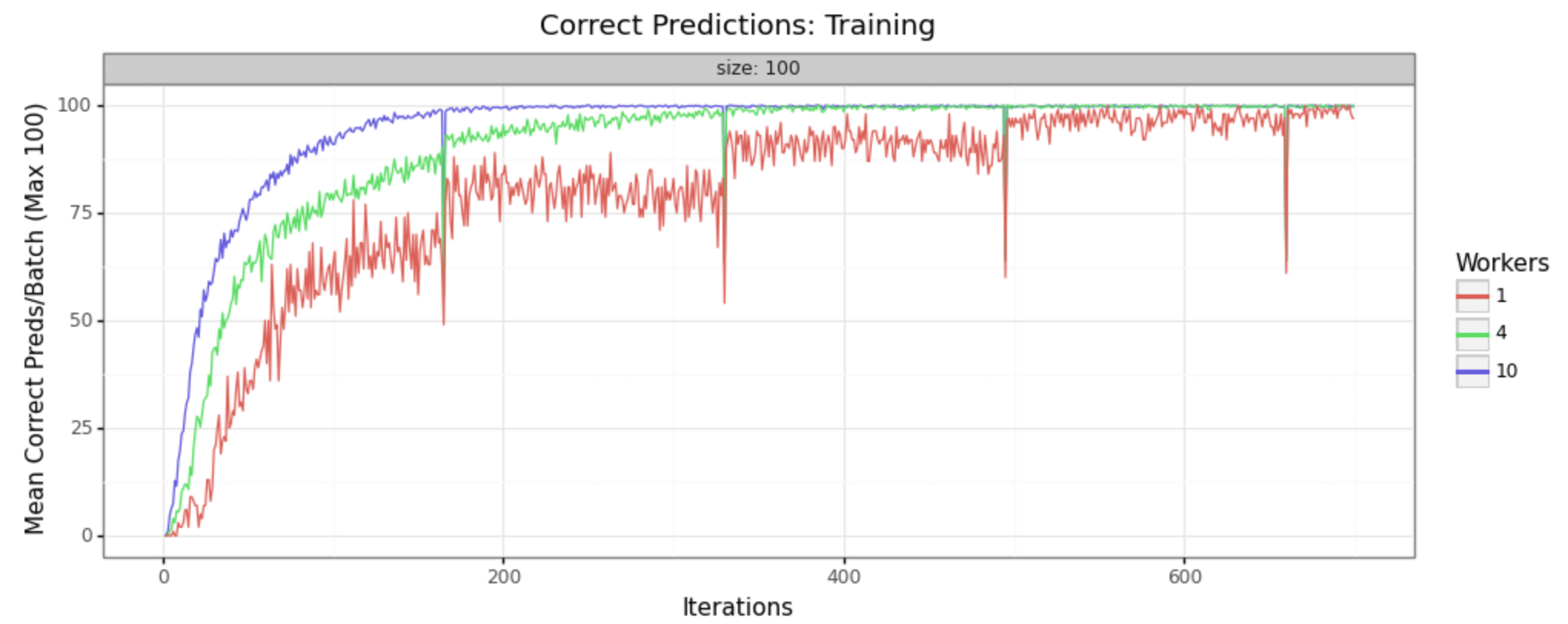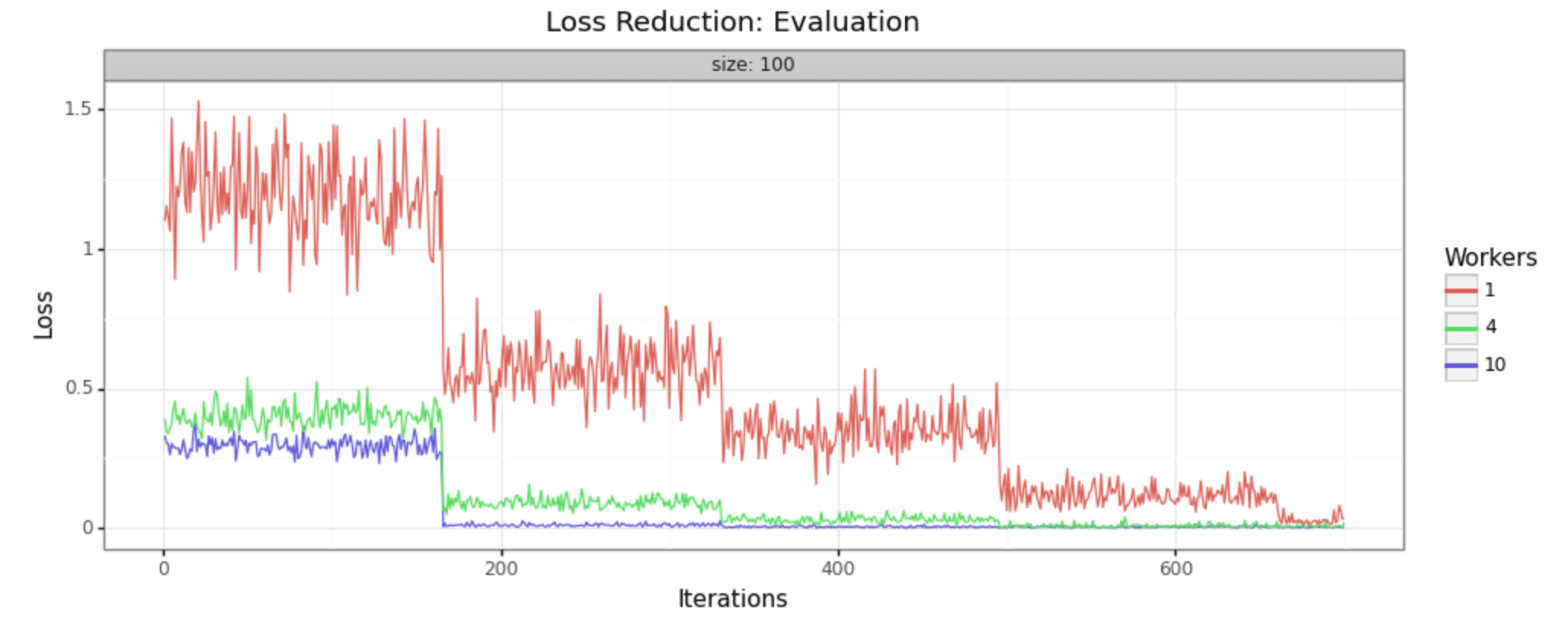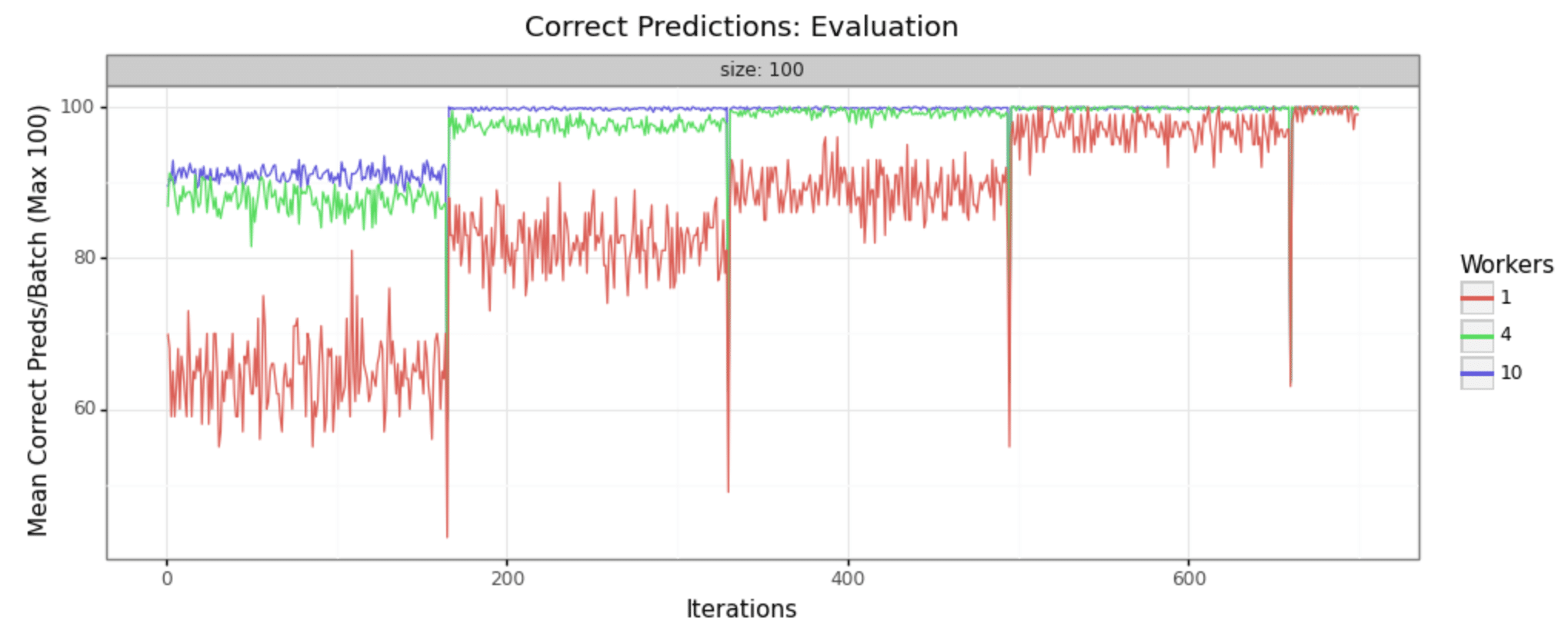# Combining Dask and PyTorch for Better, Faster Transfer Learning

## Combining Dask and PyTorch for Better, Faster Transfer Learning

### Introducing a new Python package: dask-pytorch-ddp

This tutorial is run on the Saturn Cloud platform, which makes Dask clusters available at the click of a button to users. If you need access to clusters so you can try out the steps below, we have a free version

Data parallelism within a single machine is a reasonably well-documented method for optimizing deep learning training performance, particularly in PyTorch. However, taking the step from one machine to training a single neural net on many machines at once can seem difficult and complicated.

This tutorial will demonstrate first, that GPU cluster computing to conduct transfer learning allows the data scientist to significantly improve the effective learning of a model; and second, that implementing this in Python is not as hard or scary as it sounds, especially with our new library, `dask-pytorch-ddp`

We are going to use the same dataset that we used in our PyTorch parallelized inference tutorial, the Stanford Dogs dataset. Instead of using Resnet50 as it is out of the box, we’ll improve it with transfer learning. In normal cases, this task can be very time consuming and resource-intensive, but today you are going to find out how to make it learn better and faster with parallelization.

In addition to the information provided here, we highly recommend users who are new to parallelized PyTorch visit some of the official documentation and excellent existing tutorials:

# Introducing Concepts

Before we dive into working on this, we should go over the underlying concepts around how parallelization is made possible in PyTorch.

## torch.nn.parallel.DistributedDataParallel / DDP

DistributedDataParallel is PyTorch’s native structure for parallel model training on multiple machines. There’s a lot to know about how this works, and we can’t cover it all here, but we have a summary overview to get you started.

It may help to actually start by discussing DataParallel, which is the single-machine parallelization tool that PyTorch provides. This is really enabling the same parallel training, just on a single machine, and DistributedDataParallel then extends this to be able to work on multiple GPU machines.

The official PyTorch documentation tells us this:

This container parallelizes the application of the given module by splitting the input across the specified devices by chunking in the batch dimension (other objects will be copied once per device). In the forward pass, the module is replicated on each device, and each replica handles a portion of the input. During the backwards pass, gradients from each replica are summed into the original module.

Clear as mud, right? Let’s try to break it down.

This container parallelizes the application of the given module

This is just indicating that we’re parallelizing a deep learning workflow – transfer learning in our case.

by splitting the input across the specified devices by chunking in the batch dimension

Input for a transfer learning workflow is the dataset! Ok, so it is chunking our image batches and that’s what gets to be parallel.

(other objects will be copied once per device)

Eg, our starting model, if any (Resnet50 for us) doesn’t get broken up at all. Good to know.

In the forward pass, the module is replicated on each device, and each replica handles a portion of the input.

Ok, so the training task, our module, is replicated on each device. We have multiple copies of the job working simultaneously, and each one gets a chunk of the input images rather than the entire dataset.

During the backwards pass, gradients from each replica are summed into the original module.

And then each of these duplicate tasks passes the results (the gradients) back home to the original! The learning is happening out in the workers/child processes, and then they all return the results to the original module/training process to be aggregated.

The essential difference with DDP, then, is that it is optimized for multiple machines instead of a single machine with multiple threads. It’s able to communicate across different machines effectively, so we can use a GPU cluster for our computation.

#### Still with me? It’s reasonable to find this all a little confusing!

If you are still having any trouble understanding the process, it may help to think of all our workers as individuals working on the same puzzle problem. At the end of the epoch, they all hand their findings back to the master node, which combines the partial solutions each one has submitted. Then everyone gets a copy of this combined solution, which is still not complete, and they start working on it again for another epoch. The difference is that now they have a head start thanks to everyone’s combined work.

You could just have one person doing the work, for sure – and they might eventually even reach the same overall result. But they’re going to need a lot more time to get there, and the results will be worse at the outset. The group’s progress solving the problem will be better from the get-go, because every worker is going to have a slightly different strategy for solving, so you’re getting multiple approaches combined at the same time.

We’re not necessarily creating results that would be impossible with a single node, but we’re getting better results, faster, and will be able to stop training a lot sooner.

# Taking PyTorch to the Cluster

If you’ve worked through any of our other tutorials that involve Dask clusters on Saturn Cloud, you have read a little about the commands used for instructing the client, aka our Dask cluster. We’re using that very same functionality here under the hood of `dask-pytorch-ddp` to take our distributed PyTorch job from the single worker to the cluster.

## torch.distributed.init_process_group

As the official PyTorch documentation tells us, a Process Group is required for the workers to communicate with each other and coordinate the work being completed. As a result, creating a process group is a vital first step in the setup. We have handled this for you in `dask-pytorch-ddp`, where a function called `dispatch.run` is provided, which we explain in the next section. You just supply a function that contains the PyTorch training steps and the function takes care of passing the work out to the cluster appropriately.

This particular function is pretty instrumental to the task we are undertaking, so we’ll look at it just for a moment and explain how it works. (If you are very interested in the details, the link above takes you to the full codebase for `dask-pytorch-ddp`.)

Inside this function, the client is doing a few key things:

• Producing a list of the jobs you want to run, e.g. a training task for every worker in the cluster.
• Reserving this list in memory until you indicate that computations should begin.
• Creating and destroying the Process Group as needed, so that your tasks all communicate correctly.

As a result, if you ever run into challenges regarding your cluster and its interpretation/understanding of instructions, this function may be a helpful place to start.

# See It In Action

Now that we have a general understanding of our tools, we can actually build our code to run this transfer learning task.

## Data Setup

One thing that you might realize when contemplating this problem is that loading image data from S3, as we do, might be slow – even the slowest part of our task! We thought that too, which is why we wrote an extension of the PyTorch Dataset class for this work. In `dask-pytorch-ddp` you’ll find this class named `S3ImageFolder`. This isn’t required for the workflow to operate, but it makes a huge difference in the speed at which your workflow can perform.

The arguments it requires are your S3 bucket name (string), your file prefix inside the bucket, and then any PyTorch transformations you wish to use. See below for an example of it in context. This way, any sort of file you have inside the S3 bucket can be loaded in highly parallel fashion, transformed efficiently, and then returned as a Dataset class object for use in other PyTorch tasks. We think you’ll be really impressed with the speed of processing this allows!

``````def prepro_batches(bucket, prefix):
'''Create the S3ImageFolder Dataset object, apply transformations.'''
transform = transforms.Compose([
transforms.Resize(256),
transforms.CenterCrop(250),
transforms.ToTensor()])
whole_dataset = data.S3ImageFolder(bucket, prefix, transform=transform)
return whole_dataset
``````

## Split Samples

Of course, we want to do our due diligence when training this model, so we want to create train and evaluation splits of data to ensure that the improvements we’re seeing are valid and not overfitting.

Notice that the DataLoader objects here are being explicitly defined to use multiprocessing – this means we can take full advantage of parallelization to make our image ingestion faster when we finally call it in our training job function (described below).

``````def get_splits_parallel(train_pct, data, batch_size):
'''Select two samples of data for training and evaluation'''
classes = data.classes
train_size = math.floor(len(data) * train_pct)
indices = list(range(len(data)))
np.random.shuffle(indices)
train_idx = indices[:train_size]
test_idx = indices[train_size:len(data)]

train_sampler = SubsetRandomSampler(train_idx)
test_sampler = SubsetRandomSampler(test_idx)

``````

## Setting Up Results Handling

We have one more new class to instantiate here, so that we can efficiently monitor the performance of our training task.

``````key = uuid.uuid4().hex
``````

The `DaskResultsHandler` class object has a few very useful methods, which we’ll take full advantage of. The essential purpose of this class is to organize our model’s training tasks and monitor the performance statistics for us.

One of the methods is `submit_result`. This method accepts a path (where we want results saved) and data (in our case, some JSON that tells us the current performance of the model) and handles all the work of organizing that for us.

``````rh.submit_result(
f"worker/.json",
json.dumps({'loss': loss.item(),
'learning_rate':current_lr,
'correct':correct,
'epoch': epoch,
'count': count,
'worker': worker_rank,
'sample': 'train'})
)
``````

Another of the useful methods here is `process_results`, which accepts a directory, a list of job futures, and some error handling instructions. After we have created our futures (delayed jobs assigned to workers on the cluster), we use this to formally kick off all those tasks and make computations begin. In short, this task is the last step once all our work is defined, organized, and ready to run.

``````rh.process_results(
"/home/data/parallel/ten_workers",
futures,
raise_errors=False)
``````

# Training Pipeline

This stage of the job, then, will be quite familiar to those who work in PyTorch on transfer learning or model training. We’re just going to write our model task, just as we might for single node work, and wrap it in a function so that it can be handed out to the workers.

We will look at this function in pieces first, then put it all together at the end before we run it.

## Collect Model

To prepare the model, we need to grab it from `torchvision` first and then we can pass it to the GPU compute resources. Then we’ll wrap it in `DDP` as we talked about earlier.

``````...
device = torch.device(0)
net = models.resnet50(pretrained=True)
model = net.to(device)
device_ids = 
model = DDP(model, device_ids=device_ids)
...
``````

## Set Model Parameters

Excellent. Now we can establish the regular pieces that a PyTorch model task will require- our loss function, optimizer, and learning rate scheduler.

``````...
criterion = nn.CrossEntropyLoss().cuda()
lr = base_lr * dist.get_world_size()
optimizer = optim.SGD(model.parameters(), lr=lr, momentum=0.9)
scheduler = lr_scheduler.ReduceLROnPlateau(optimizer, mode = 'min', patience = 2)
...
``````

You may notice I am choosing a learning rate scheduler that waits for plateau of the loss function before shifting – this is a matter of preference, and you could certainly use a step learning rate scheduler (`StepLR`) here with no ill effects. It’s a matter of what works best for your data and base model.

## Retrieve Data from S3 and Process

Now, we collect our data. We need to initialize the data loader objects, using our `S3ImageFolder` class and the train/test splits, and name our data loaders for later reference. The DataLoader class allows us to lazily load the images when our training loop is ready for them – a major asset for this work.

``````...
whole_dataset = prepro_batches(bucket, prefix)
train, val = get_splits_parallel(train_pct, whole_dataset, batch_size=batch_size)
dataloaders = {'train' : train, 'val': val}
...
``````

## Training Iterations

At this point, we’re ready to begin iteration over the number of epochs we have chosen. Here we set the model to training mode, and loop over the batches of images that our “train” DataLoader is referencing from S3.

``````...
count = 0
t_count = 0
for epoch in range(n_epochs):
model.train()  # Set model to training mode
dt = datetime.datetime.now().isoformat() #used later for tracking results
inputs = inputs.to(device)
labels = labels.to(device)

outputs = model(inputs)
_, preds = torch.max(outputs, 1)
loss = criterion(outputs, labels)
correct = (preds == labels).sum().item()

loss.backward()
optimizer.step()
count += 1
...
``````

This isn’t the end of our loop, however. We have the `DaskResultsHandler` methods to collect statistics about each iteration and to checkpoint our model performance at appropriate intervals. We know the learning rate, loss, and count of correct predictions from this batch, and so we will write all that out along with “count” which is what number this iteration happens to be.

``````...
for param_group in optimizer.param_groups:
current_lr = param_group['lr']
# Record the results of this model iteration (training sample) for later review.
rh.submit_result(
f"worker/.json",
json.dumps({'loss': loss.item(),
'learning_rate':current_lr,
'correct':correct,
'epoch': epoch,
'count': count,
'worker': worker_rank,
'sample': 'train'})
)

if (count % 100) == 0 and worker_rank == 0:
# Grab a snapshot of the current state of the model, in case of interruption or need to review
rh.submit_result(
f"checkpoint-.pkl",
pickle.dumps(model.state_dict())
)

# Adjust the learning rate based on training loss
scheduler.step(loss)
...
``````

## Evaluation Iterations

At this point, we have the complete function allowing us to train the model! Of course we also need evaluation steps to validate our statistics, so we’ll add a second chunk (still within the same epoch) to do that.

``````...
model.eval()  # Set model to evaluation mode
dt = datetime.datetime.now().isoformat()
inputs_t = inputs_t.to(device)
labels_t = labels_t.to(device)

outputs_t = model(inputs_t)
_,pred_t = torch.max(outputs_t, dim=1)
loss_t = criterion(outputs_t, labels_t)
correct_t = (pred_t == labels_t).sum().item()
t_count += 1

# statistics
for param_group in optimizer.param_groups:
current_lr = param_group['lr']
# Record the results of this model iteration (evaluation sample) for later review.
rh.submit_result(
f"worker/.json",
json.dumps()
)
...
``````

This completes the workflow – we have all we need to pass to each worker to have parallelized training!

## Put it all together

``````def run_transfer_learning(bucket, prefix, train_pct, batch_size, n_epochs, base_lr):
and run transfer learning over n epochs.'''
worker_rank = int(dist.get_rank())

# Format model and params
device = torch.device(0)
net = models.resnet50(pretrained=True)
model = net.to(device)
device_ids = 
model = DDP(model, device_ids=device_ids)

criterion = nn.CrossEntropyLoss().cuda()
lr = base_lr * dist.get_world_size()
optimizer = optim.SGD(model.parameters(), lr=lr, momentum=0.9)
scheduler = lr_scheduler.ReduceLROnPlateau(optimizer, mode = 'min', patience = 2)

# Retrieve data for training and eval
whole_dataset = prepro_batches(bucket, prefix)
train, val = get_splits_parallel(train_pct, whole_dataset, batch_size=batch_size)

# Prepare metrics aggregation
count = 0
t_count = 0
for epoch in range(n_epochs):
# Each epoch has a training and validation phase
model.train()  # Set model to training mode
dt = datetime.datetime.now().isoformat()
inputs = inputs.to(device)
labels = labels.to(device)

outputs = model(inputs)
_, preds = torch.max(outputs, 1)
loss = criterion(outputs, labels)
correct = (preds == labels).sum().item()

loss.backward()
optimizer.step()
count += 1
# statistics
for param_group in optimizer.param_groups:
current_lr = param_group['lr']
# Record the results of this model iteration (training sample) for later review.
rh.submit_result(
f"worker/.json",
json.dumps()
)

if (count % 100) == 0 and worker_rank == 0:
# Grab a snapshot of the current state of the model, in case of interruption or need to review
rh.submit_result(f"checkpoint-.pkl", pickle.dumps(model.state_dict()))

model.eval()  # Set model to evaluation mode
dt = datetime.datetime.now().isoformat()
inputs_t = inputs_t.to(device)
labels_t = labels_t.to(device)

outputs_t = model(inputs_t)
_,pred_t = torch.max(outputs_t, dim=1)
loss_t = criterion(outputs_t, labels_t)
correct_t = (pred_t == labels_t).sum().item()
t_count += 1

# statistics
for param_group in optimizer.param_groups:
current_lr = param_group['lr']
# Record the results of this model iteration (evaluation sample) for later review.
rh.submit_result(
f"worker/.json",
json.dumps()
)

scheduler.step(loss)
``````

It seems like a lot, but once we have discussed it piece-by-piece it is really not much different than any other training workflow. However, don’t forget that this is all just a function still! We are holding it all in suspended animation until we’re ready to actually kick off parallel work on the cluster.

The final job is to pass this to our cluster, which we can do with just a couple of lines. Remember that earlier we discussed the method on `DaskResultsHandler` called `process_results()` which will retrieve the futures that are being calculated on the workers.

Create the futures first…

``````startparams = {'n_epochs': 5,
'batch_size': 100,
'train_pct': .8,
'base_lr': 0.01}

futures = dispatch.run(
client,
run_transfer_learning,
prefix = "dogs/Images",
**startparams)
``````

… then set the computations off!

``````rh.process_results(
"/home/jovyan/stats/parallel/pt8_10wk",
futures,
raise_errors=False)
``````

# Exploring Results

In order to demonstrate that this methodology is, in fact, producing improvements in model training, it can help to look at some visual representations of the statistics collected. We have run the job with three different sizes of cluster, to make it easy to see- 1 worker (single node), 4 workers, and 10 workers.

We can in fact see very noticeable performance gains in both loss reduction and accuracy. Batch size is 100 for each, and the adaptive learning rate begins at .01 for each. The train/test split is 80/20, as shown in the code above.

### Training Samples### Evaluation SamplesAs you can see, increasing the number of workers in our cluster markedly improves the performance of training. In a 10-worker cluster, we reach peak performance around 200 iterations, while with the 4-worker cluster we must wait til 400 or more. In the single node example, at 600 we still have substantial noise in the loss value and may not reach the desired performance for many iterations to come.

# Conclusion

It depends tremendously on the individual problem being solved whether GPU clusters for transfer learning are the right choice, and some problems are not complex or challenging enough to call for this approach. However, for many deep learning problems, especially in the computer vision space, there can be a substantial value generated by using the increased computation resources and speeding up the achievement of ideal model performance.

For data scientists who would like to have faster, better performance on transfer learning and deep learning modeling tasks, we encourage you to give GPU clusters on Saturn Cloud a try! You can use our free version to experiment and see if this approach is right for your problem.

Thanks to Alvan Nee on Unsplash for the header image.